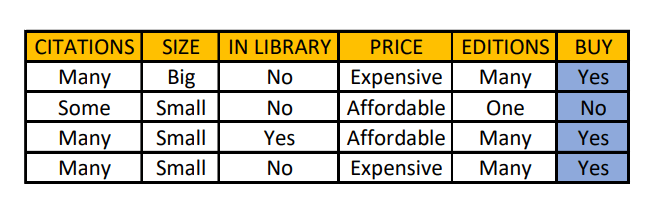### Introduction

In Concept Learning, whenever we search through a predefined space of potential hypotheses in order to find the version space from training examples in a binary classification problem, then the Candidate Elimination Algorithm is one of the best algorithms that can be used to generate the required version space of hypotheses.

The Candidate Elimination Algorithm represents the version space by storing only its most general members G and its most specific members S. Given only these two sets S and G, it is possible to enumerate all members of a version space by generating hypotheses that lie between these two sets in general-to-specific partial ordering over hypotheses. Every member of the version space lies between these boundaries.

### What is Version Space?

Version space represents the set of all hypotheses in H, consistent with the observed training examples, D. Finding out the version space for a set of training examples is better than finding out a single hypothesis as in the Find-S algorithm. This is because there is a possibility of the existence of more hypotheses in H that are consistent with the dataset, which is probably present in a more generalized form than the one we have inferred from the Find-S algorithm. A version space can be represented with its general and specific boundary sets.

### Algorithm

Here are the steps that need to be followed in order to generate the version space using Candidate Elimination Algorithm:

• Initialize G to the set of maximally general hypotheses in H.
• Initialize S to the set of maximally specific hypotheses in H
• For each training example ‘d’:
• If d is a positive example, then generalize the specific hypothesis (S).
• Else make the general hypothesis (G) more specific.
• Find the version space from S and G.

Note: Most specific hypothesis contains ‘ϕ’ for all the label attributes involved with the data while the most general hypothesis contains ‘?’.

### Inference from the Version Space

Let us consider an example. The data given below shows the record of the purchase of various books by a person. Using the Candidate Elimination Algorithm, we can find the version space and infer whether this person will buy a particular book in the future or not.Initially,

Specific Hypothesis S = <ϕ,ϕ,ϕ,ϕ,ϕ>

General Hypothesis G = <?,?,?,?,?>

Itemset 1: Label = Yes -> Generalize S

S = <Many, Big, No, Expensive, Many>

G = <?,?,?,?,?>

Itemset 2: Label = No-> Specialize G

S = <Many, Big, No, Expensive, Many>

G = { <Many, ?, ?, ?> , <?, Big, ?, ?, ?>, <?, ?, ?, Expensive, ?>, <?, ?, ?, ?, Many> }

Note: The specialization of G takes place for only one column at a time. Therefore, G becomes a set of hypotheses after specialization.

Itemset 3: Label = Yes -> Generalize S

S = <Many, ?, ?, ?, Many>

G = { <Many, ?, ?, ?>, <?, ?, ?, ?, Many> }

Note: Whenever we generalize the specific hypothesis S, we need to ensure that the general hypothesis G is in-sync with the changes in S. We eliminate all the specialized hypotheses from G if those attributes have been generalized in S.

Itemset 4: Label =  Yes -> Generalize S

S = <Many, ?, ?, ?, Many>

G = { <Many, ?, ?, ?>, <?, ?, ?, ?, Many> }

What does this version space infer?

The version gives us all the possible hypotheses that can yield a correct prediction for our label of interest.

### Implementation

Let us build a program that computes the general and specific hypothesis according to the Candidate Elimination algorithm.

				
import java.util.Arrays
val data = arrayOf(
arrayOf("Sunny", "Warm", "Normal", "Strong", "Warm", "Same", "Yes"),
arrayOf("Sunny", "Warm", "High", "Strong", "Warm", "Same", "Yes"),
arrayOf("Sunny", "Warm", "Normal", "Strong", "Warm", "Same", "Yes"),
arrayOf("Sunny", "Warm", "High", "Strong", "Cool", "Change", "Yes"),
arrayOf("Rainy", "Cold", "High", "Strong", "Warm", "Change", "No"))
val g = arrayOfNulls(data.size - 1)
val s = arrayOfNulls(data.size - 1)
for (i in 0 until data.size - 1)
{
s[i] = "ϕ"
g[i] = "?"
}
var flag = true
System.out.println("General hypothesis: ")
for (i in data.indices)
{
if (data[i][s.size] === "Yes")
{
if (flag == true)
{
for (j in s.indices)
{
s[j] = data[i][j]
}
flag = false
continue
}
for (j in s.indices)
{
if (s[j] === "?")
{
continue
}
else if (s[j] === data[i][j])
{
continue
}
else if (s[j] !== data[i][j])
{
s[j] = "?"
continue
}
}
}
for (j in g.indices)
{
if (s[j] === "?")
{
continue
}
else if (s[j] !== data[i][j])
{
val temp = g[j]
g[j] = s[j]
System.out.println(Arrays.toString(g))
g[j] = temp
continue
}
else if (s[j] === data[i][j])
{
continue
}
}
}
System.out.println("Specific hypothesis: " + Arrays.toString(s))



Output:

				
General Hypotheis:
[Sunny, ?, ?, ?, ?, ?]
[?, Warm, ?, ?, ?, ?]
Specific hypothesis: [Sunny, Warm, ?, Strong, ?, ?]